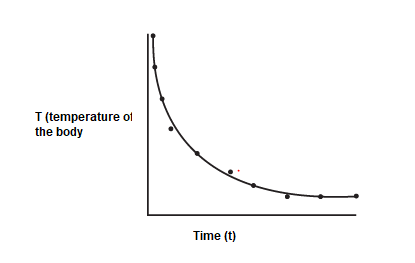# Newton's Law of Cooling

## Newton's Law of Cooling

• A hot body loses heat to its surroundings in the form of heat radiation. The rate of loss of heat depends on the difference in temperature between the body and its surroundings. Newton was the first to study, in a systematic manner, the relation between the heat lost by a body in a given enclosure and its temperature.
• Consider a hot body at temperature T1 is placed in surrounding at temperature T2. and let T be the temperature of the body at time t
• For small temperature difference between the body and surrounding rate of cooling is directly proportional to the temperature difference and surface area exposed i.e.,
$\frac {dT}{dt} = -bA (T - T_2)$ ---(1)
• This is known a Newton's law of cooling.
b depends on nature of surface involved and the surrounding conditions. Negative sign is to indicate that $T > T_2$ , $\frac {dT}{dt}$ is negative and temperature decreases with time
• According to this law, the rate of cooling is directly proportional to the excess of temperature.
• Arranging the equation (1) as
$\frac {dT}{T - T_2} =-bAdt$
On integrating
$log_e (T -T_2) =-kt + C$
or
$T - T_2 = e^{-kt + C}$
$T = T_2 + e^{-kt + C}$
Applying initial condition t=0
$e^c= T_1 - T_2$
So , the final equation will be
$T =T_2 + (T_1 -T_2)e^{-kt}$
Now $t -> \infty$, $T=T_2$
i,e as the time goes, body temperature becomes equal to the surrounding temperature
• The graph between Temperature of the body and time is shown below. It is called Newton law of cooling curve. The slope of the graph gives the the rate of cooling of the body## Method of Solving problems on Newton's Law of Cooling

Method -1
For exact calculation, it is recommended to use the below equation
$T =T_2 + (T_1 -T_2)e^{-kt}$

Where
$T_2$ -> Surrounding temperature
$T$-> Body temperature at time t
$T_1$ -> Initial temperature of the body
k-> constant
t-> Time
Method -2
For approximate calculation , we can use the newton law of cooling as
$\frac {dT}{dt} = k (T -T_2)$
$\frac {T_i - T_f}{t} = k(T - T_2)$
Where
$T_i$-> Initial temperature
$T_f$ -> Final temperature
t-> time taken
T -> Average temperature of the body in the time interval i.e $\frac {T_f + T_i}{2}$
$T_2$ -> Surrounding temperature

## Solved Example

Question 1 A pan filled with hot food cools from 94 °C to 86 °C in 2 minutes when the room temperature is at 20 °C. How long will it take to cool from 71 °C to 69 °C?
Solution
Using the approximation method
For first temperature decrease
$\frac {8}{2} = k( \frac {94+86}{2} - 20)$
$4=70 k$ -(1)
Now for second temperature decrease
$\frac {2}{t} = k( \frac {71+69}{2} - 20$
$\frac {2}{t}=50 k$ -(2)
From (1) and (2), we have
t=.7 min